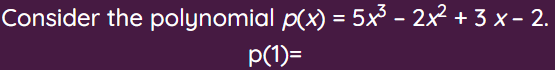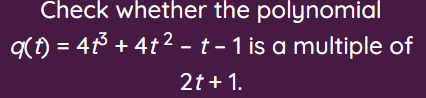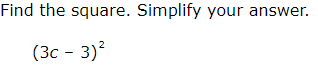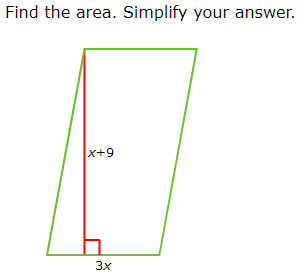# Polynomials and its Applications

This set of MCQs helps you brush up on important math topics and prepare you to dive into skill practice.

Start Quiz4

-3

-4

2

Find a zero of the polynomial p(x) = 2x + 1.

2

1/2

-1/2

-2

Find the remainder obtained on dividing p(x) = x^3 + 1 by x + 1.

2

-1

0

1

Factorize. y^2 +5y -6

(y+1)(y-6)

(y+2)(y-3)

(y+6)(y-1)

(y+1)(y-12)Yes

No

Cannot be determined

None of these-9c^2–18c+9

9c^2+18c+9

9c^2–18c-9

9c^2–18c+90

3x^2+27x

3x^2-27x

27x^2+3x

Quiz/Test Summary
Title: Polynomials and its Applications
Questions: 7
Contributed by: